## Simulation of a scattering curve from a cylindrical core-shell model

In the following we calculate the scattering curve of a cylindrical shell model with an axially inhomogeneous (layered) core which might be a possible structural model for the LDL-molecule. The dimensions, radial and axial electron densities are shown in the following picture (cylindrical core-shell model with an axial cut through the cylindrical shell) below: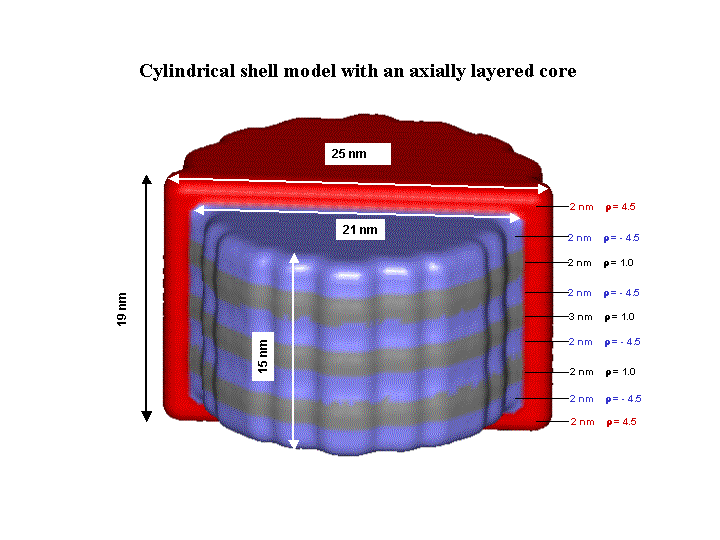The parameter for this model are entered as input into the scattering curve simulator as shown below: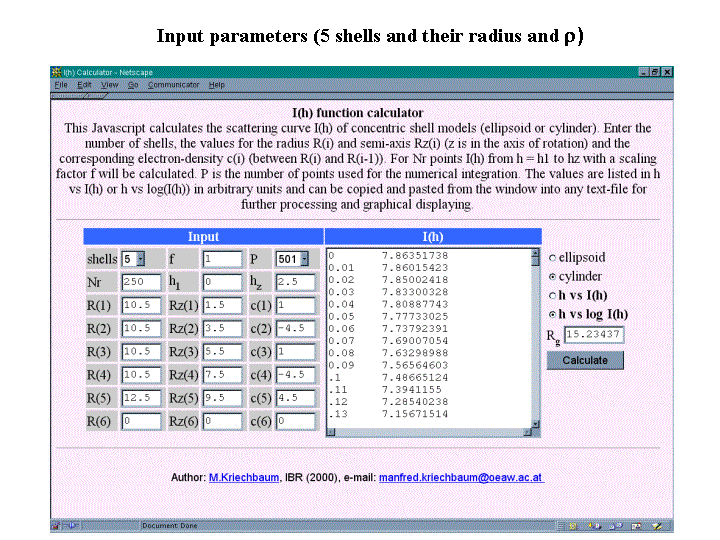The resulting scattering curve I(h) in a lin-log plot from h = 0 to 2.5 [1/nm]: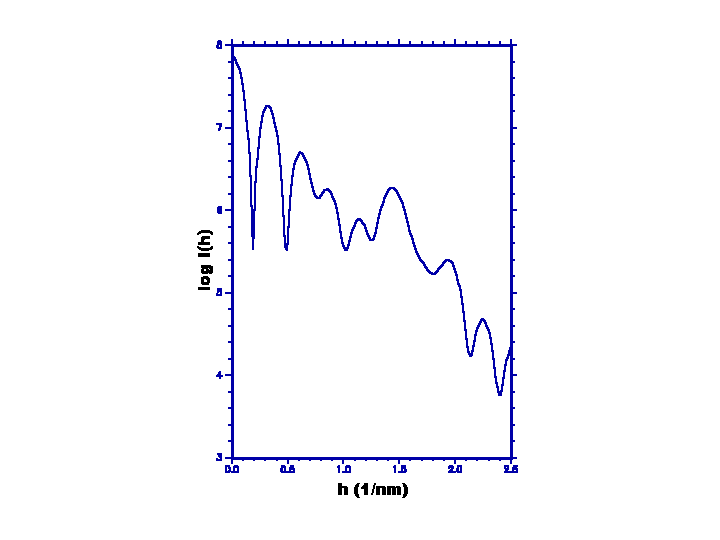The p(r)-function (Dmax = 31.4 nm) was calculated from this scattering curve using the program GIFT (O.Glatter):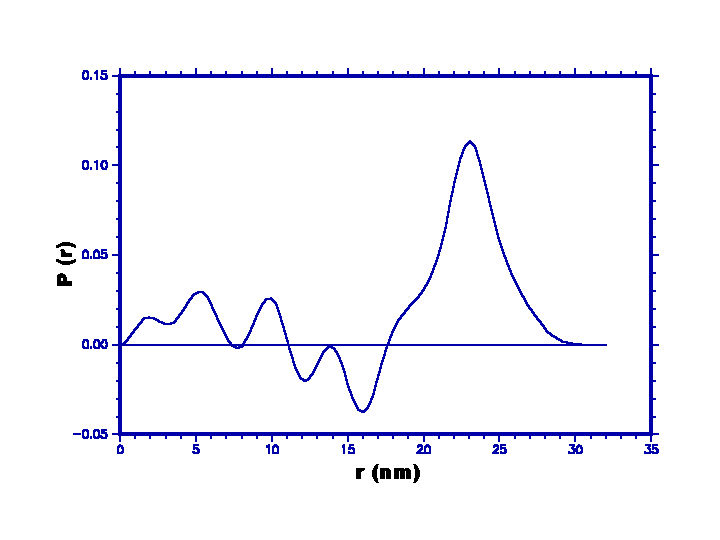For comparison the I(h) and p(r) functions were also calculated using the program SINGLEBODY (G.Fritz):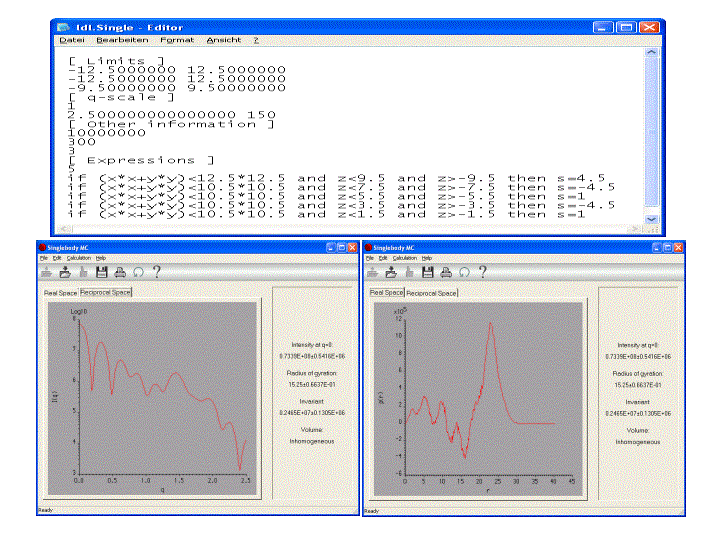Author: Manfred Kriechbaum manfred.kriechbaum@tugraz.at (Sept. 25th 2002).